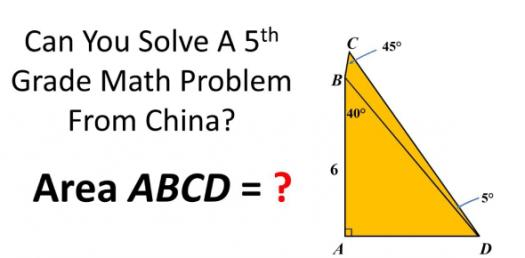# 5th Grade Math Quiz: Basic Word Problems! Trivia Questions

Approved & Edited by ProProfs Editorial Team
At ProProfs Quizzes, our dedicated in-house team of experts takes pride in their work. With a sharp eye for detail, they meticulously review each quiz. This ensures that every quiz, taken by over 100 million users, meets our standards of accuracy, clarity, and engagement.
| Written by Efrain_vr
E
Efrain_vr
Community Contributor
Quizzes Created: 1 | Total Attempts: 358
Questions: 5 | Attempts: 363SettingsHere is a 5th Grade Math Quiz made up of Basic Word Problems! These types of math problems are perfect for activating your mind in the morning and keeping you on your toes when it comes to calculations. How about you give it a try and get to see how well you will do? All the best as you tackle it!

• 1.

### Adam is 4.5 feet tall. He is 0.75 foot taller than his brother Mark. How tall is Mark?

• A.

3.25 ft

• B.

4.25 ft

• C.

3.75 ft

• D.

5.25 ft

C. 3.75 ft
Explanation
Based on the given information, Adam is 0.75 feet taller than Mark. If Adam's height is 4.5 feet, then Mark's height would be 4.5 - 0.75 = 3.75 feet. Therefore, Mark is 3.75 feet tall.

Rate this question:

• 2.

### An employee at a video store worked a total of 90 hours in 3 weeks. She worked 5 days a week. If she worked the same number of hours a day, how many hours did the employee work each day?

• A.

8

• B.

6

• C.

9

• D.

12

B. 6
Explanation
The employee worked a total of 90 hours in 3 weeks. To find out how many hours she worked each day, we need to divide the total number of hours by the number of days she worked. Since she worked 5 days a week, we divide 90 by 15 (3 weeks x 5 days per week). This gives us 6, so the employee worked 6 hours each day.

Rate this question:

• 3.

### Mr. Cantú will put 1 flag on each table in the cafeteria for a school event. The cafeteria has 15 rows with 5 tables in each row and 12 rows with 4 tables in each row. Mr. Cantú already has 94 flags. How many more flags does he need to buy?

• A.

48

• B.

19

• C.

27

• D.

29

D. 29
Explanation
Mr. Cantú needs to put a flag on each table in the cafeteria, and there are a total of 15 rows with 5 tables in each row, which gives a total of 15 * 5 = 75 tables. Additionally, there are 12 rows with 4 tables in each row, which gives a total of 12 * 4 = 48 tables. Therefore, the total number of tables in the cafeteria is 75 + 48 = 123. Mr. Cantú already has 94 flags, so he needs to buy 123 - 94 = 29 more flags.

Rate this question:

• 4.

### Connie is preparing for a spelling bee. She wants to study the same number of words each week. The table below shows the number of words she plans to have studied by the end of different numbers of weeks.  Connie’s Study Plan    Week  Total Number of Words 1 20 3 60 7 140 9 180   Based on this information, which of the following statements is true?

• A.

The total number of words studied will be 20 times the number of weeks.

• B.

The total number of words studied will be 20 more than the number of weeks.

• C.

The total number of words studied will be 40 more than the number of weeks.

• D.

The total number of words studied will be 40 times the number of weeks.

A. The total number of words studied will be 20 times the number of weeks.
Explanation
The total number of words studied will be 20 times the number of weeks because the number of words studied doubles each week. For example, in week 3, Connie plans to have studied 60 words, which is 20 words more than in week 1. Similarly, in week 7, she plans to have studied 140 words, which is 40 words more than in week 3. This pattern continues, indicating that the total number of words studied will always be 20 times the number of weeks.

Rate this question:

• 5.

### Reba is planning a dinner for 24 people. She wants to make 3 tacos for each person. Taco shells are sold in packages of 12. Which of the following equations can be used to find p, the number of packages of taco shells needed for the dinner?

• A.

(24 ×3) ÷12 = p

• B.

(24 ÷3) ×12 = p

• C.

(24 ×3) ×12 = p

• D.

(24 ÷3) +12 = p

A. (24 ×3) ÷12 = p
Explanation
To find the number of packages of taco shells needed for the dinner, we need to calculate the total number of tacos required. Since Reba wants to make 3 tacos for each person and she is planning a dinner for 24 people, the total number of tacos needed will be 24 multiplied by 3, which is 72.

Since taco shells are sold in packages of 12, we can divide the total number of tacos needed by 12 to find the number of packages required. Therefore, the equation (24 × 3) ÷ 12 = p can be used to find the number of packages of taco shells needed for the dinner.

Rate this question:

Related TopicsBack to top
×

Wait!
Here's an interesting quiz for you.